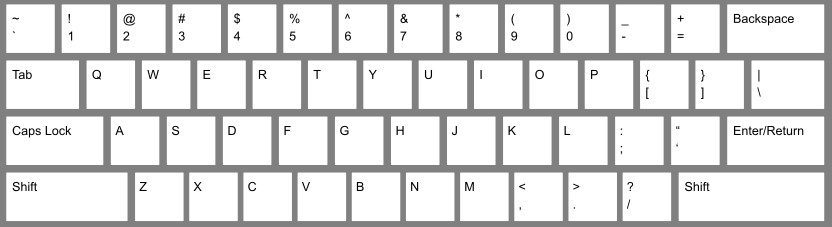# Some Math Basics

There are many fields in Mathematics, the most commonly thought of fields are Algebra, Geometry, and Statistics. Even these fields have more depth to them than the average person will use, but they all have some interesting bits. Some of the less well known, but equally important topics include Set Theory, Game Theory, Logic, and many others. I do not claim to be an expert on any of the major topics, however, I do teach many topics in different portions of the great field of Mathematics.

Some tools we will need for these include symbols and definitions. Some symbols are used in more than one way through the different parts of Math, when this happens, the meaning will be refreshed at that time. There are some symbols with the same meaning through all parts of Math. These are in the table below. Most symbols are on the standard American keyboard, below from Wikipedia.

Other symbols will be added later with links to the post that explains the use.

 Symbol Name Meaning Example x, y, z usually a variable a, b, c, n, m usually a constant any real number but does not change Pi Represents the ratio of a Circle’s circumference to its diameter. + Plus Add the two terms together, may also be used for a positive integer 3 + 2, +4 – Minus Subtract the two terms, may also be used for a negative integer 4 – 7, -3 x, * Multiplication sign Multiply the two terms, the x is not used when it could be confused with the variable, I normally use *, except in certain cases. The symbol may also not be written in, in certain cases. 3 x 2, 4x * 8 ٪, / Division sign Divide the two terms, several ways to be written, will be discussed in detail in a different post. 9 ٪ 3, 8/2 = Equals Left & right sides are the same a = 3, 2 = 2 < Less than Left side is less than the right side 2 < 5 , <= Less than or equal to > Greater than Left side is greater than the right side 7 > 3 , >= Greater than or equal toKeyboard layout image from: https://en.wikipedia.org/wiki/Keyboard_layout#/media/File:ANSI_Keyboard_Layout_Diagram_with_Form_Factor.svg

Save